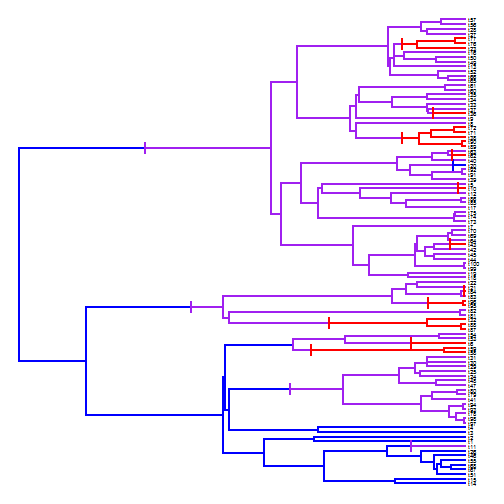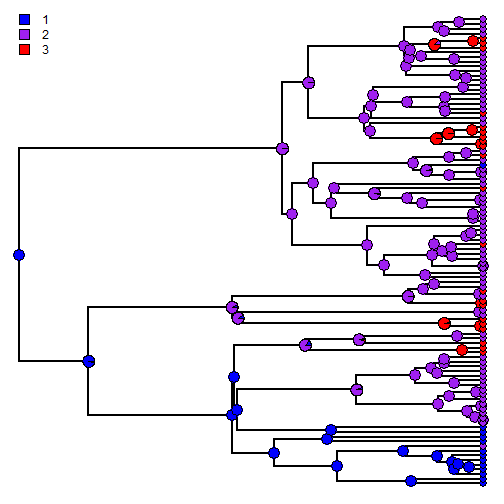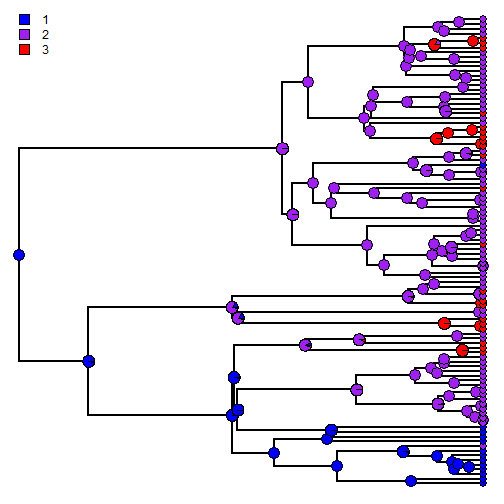## Sunday, November 1, 2015

### Stochastic mapping with an ordered character

Yesterday I received the following question:

I have three character states (1,2,3) and Iâ€™d like to create stochastic maps for a situation where character change is constrained to go through state 2, so that the 1->3 and 3->1 transitions are not allowed. In my analyses so far Iâ€™ve just been using and ARD model, but I wondered if there is a way to implement these constrained pathways so that the Q matrix only allows certain specified transitions? I was also wondering if there is an easy way to constrain the root state for the analysis?

Both of these things are pretty easy to do. Here, I'll demonstrate with simulated data & tree:

``````library(phytools)
tree
``````
``````##
## Phylogenetic tree with 100 tips and 99 internal nodes.
##
## Tip labels:
##  t14, t15, t51, t67, t68, t55, ...
##
## Rooted; includes branch lengths.
``````
``````x
``````
``````##  t14  t15  t51  t67  t68  t55  t46  t26  t11   t1   t2   t3   t4  t97  t98
##  "1"  "1"  "1"  "1"  "1"  "1"  "1"  "1"  "2"  "1"  "1"  "1"  "1"  "2"  "2"
##  t78  t93  t94  t41  t79  t80  t47  t48  t24  t25  t29  t30  t31  t58  t59
##  "2"  "2"  "2"  "2"  "2"  "2"  "2"  "2"  "2"  "2"  "2"  "2"  "2"  "3"  "3"
##   t6  t53  t54  t87  t88  t32  t81  t82  t95  t96  t83  t84  t21  t22  t18
##  "3"  "2"  "2"  "3"  "3"  "3"  "2"  "2"  "3"  "3"  "2"  "3"  "2"  "2"  "2"
##  t19  t99 t100  t44  t45  t42  t43  t64  t69  t70   t7  t73  t74  t75  t17
##  "2"  "2"  "2"  "2"  "2"  "2"  "3"  "2"  "2"  "2"  "2"  "2"  "2"  "2"  "2"
##  t85  t86  t12  t10   t5  t39  t91  t92  t20  t40  t62  t63  t89  t90  t38
##  "2"  "2"  "2"  "3"  "2"  "2"  "2"  "2"  "1"  "2"  "3"  "2"  "3"  "3"  "3"
##  t71  t72   t8   t9  t36  t37  t33  t34  t35  t60  t61  t65  t66  t52  t13
##  "3"  "3"  "2"  "2"  "3"  "2"  "2"  "2"  "2"  "2"  "2"  "2"  "2"  "2"  "2"
##  t49  t50  t16  t23  t76  t77  t27  t28  t56  t57
##  "2"  "2"  "2"  "3"  "3"  "3"  "2"  "2"  "2"  "2"
``````

First, let's make the model we want to fit. Here, I assume that there is two rates - one of forward transition, and a second of backward transition- but I could have also fit a model with a single rate, or one in which each type of permitted change had a different rate. (This just depends on my preference.)

``````model<-matrix(c(0,1,0,2,0,1,0,2,0),3,3)
rownames(model)<-colnames(model)<-1:3
model
``````
``````##   1 2 3
## 1 0 2 0
## 2 1 0 2
## 3 0 1 0
``````
``````prior<-setNames(c(1,0,0),1:3)
prior
``````
``````## 1 2 3
## 1 0 0
``````

OK, now we are ready to do our stochastic mapping:

``````trees<-make.simmap(tree,x,model=model,pi=prior,nsim=100)
``````
``````## make.simmap is sampling character histories conditioned on the transition matrix
##
## Q =
##            1          2          3
## 1 -1.0920856  1.0920856  0.0000000
## 2  0.1074346 -1.1995202  1.0920856
## 3  0.0000000  0.1074346 -0.1074346
## (estimated using likelihood);
## and (mean) root node prior probabilities
## pi =
## 1 2 3
## 1 0 0
``````
``````## Done.
``````

First, here is our result:

``````par(mfrow=c(10,10))
colors<-setNames(c("blue","purple","red"),1:3)
plot(trees,lwd=1,ftype="off",colors=colors)
``````We can also do any specific tree:

``````plot(trees[],colors=colors,fsize=0.5)
markChanges(trees[],colors=colors)
``````Or summarize our results across trees:

``````obj<-summary(trees)
obj
``````
``````## 100 trees with a mapped discrete character with states:
##  1, 2, 3
##
## trees have 18.27 changes between states on average
##
## changes are of the following types:
##       1,2 1,3  2,1   2,3 3,1 3,2
## x->y 5.63   0 1.19 11.25   0 0.2
##
## mean total time spent in each state is:
##              1          2         3    total
## raw  4.3721937 11.2234788 1.7854335 17.38111
## prop 0.2515486  0.6457287 0.1027227  1.00000
``````
``````plot(obj,colors=colors,ftype="off")
``````Finally, it is also possible to use Bayesian MCMC to sample the parameters of the transition process from their posterior distribution rather than setting them to their ML values (as is the default). This otherwise is done in a very similar way to the above. For instance:

``````trees.mcmc<-make.simmap(tree,x,model=model,pi=prior,Q="mcmc",nsim=100)
``````
``````## Running MCMC burn-in. Please wait....
## Running 10000 generations of MCMC, sampling every 100 generations.
##
## make.simmap is simulating with a sample of Q from
## the posterior distribution
##
## Mean Q from the posterior is
## Q =
##            1          2          3
## 1 -1.0707285  1.0707285  0.0000000
## 2  0.2334955 -1.3042240  1.0707285
## 3  0.0000000  0.2334955 -0.2334955
## and (mean) root node prior probabilities
## pi =
## 1 2 3
## 1 0 0
``````
``````## Done.
``````
``````obj<-summary(trees.mcmc)
plot(obj,colors=colors,ftype="off")
``````(Note that for this to work is written you will need a bug fix for `make.simmap` that can be obtained by installing from GitHub.)

BTW, these were done on simulated data. Simulation code was as follows:

``````library(phytools)
Q<-matrix(c(-1,1,0,0.2,-1.2,1,0,0.2,-0.2),3,3)
rownames(Q)<-colnames(Q)<-1:3
tree<-pbtree(n=100,scale=1)
x<-sim.history(tree,t(Q),anc="1")\$states
``````

That's all for now.

1.This comment has been removed by the author.

2.Dear Liam, thank you for your work!
I have a similar situation, the character states are 0, 1 and the change is allow only for 0->1 and not vice versa. Furthermore the prior is the state 0. I build the model like this:

> model<-matrix(c(0,0,1,0),2,2)
> rownames(model)<-colnames(model)<-0:1
> model
## 0 1
#0 0 0
#1 1 0

and set the prior
> prior<-setNames(c(1,0),0:1)

#0 1
#1 0

but I recived this error when I did the stochastic mapping
> trees<-make.simmap(tree,x,model=model,pi=prior,nsim=100,Q="mcmc")